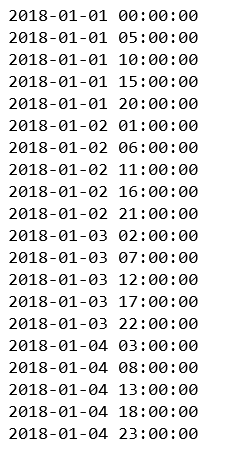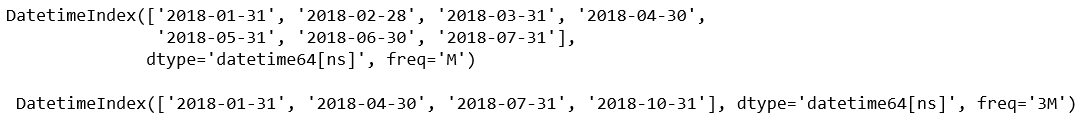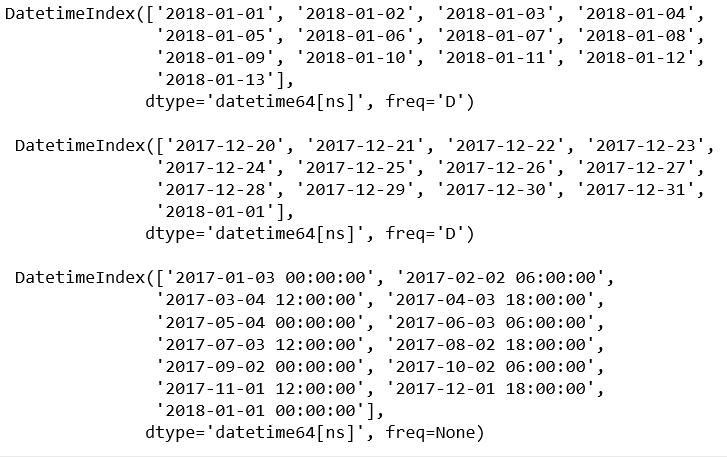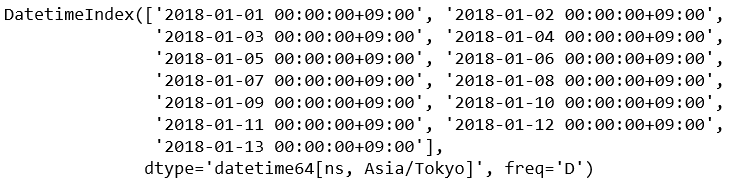# Python | pandas.date_range() method

Python is a great language for doing data analysis, primarily because of the fantastic ecosystem of data-centric python packages. Pandas is one of those packages and makes importing and analyzing data much easier.

`pandas.date_range()` is one of the general functions in Pandas which is used to return a fixed frequency DatetimeIndex.

Syntax: pandas.date_range(start=None, end=None, periods=None, freq=None, tz=None, normalize=False, name=None, closed=None, **kwargs)

Parameters:
start : Left bound for generating dates.
end : Right bound for generating dates.
periods : Number of periods to generate.
freq : Frequency strings can have multiples, e.g. ‘5H’. See here for a list of frequency aliases.
tz : Time zone name for returning localized DatetimeIndex. By default, the resulting DatetimeIndex is timezone-naive.
normalize : Normalize start/end dates to midnight before generating date range.
name : Name of the resulting DatetimeIndex.
closed : Make the interval closed with respect to the given frequency to the ‘left’, ‘right’, or both sides (None, the default).

Returns: DatetimeIndex

Code #1:

 `# importing pandas as pd ` `import` `pandas as pd ` ` `  `per1 ``=` `pd.date_range(start ``=``'1-1-2018'``,  ` `         ``end ``=``'1-05-2018'``, freq ``=``'5H'``) ` ` `  `for` `val ``in` `per1: ` `    ``print``(val) `

Output:Code #2:

 `# importing pandas as pd ` `import` `pandas as pd ` ` `  `dRan1 ``=` `pd.date_range(start ``=``'1-1-2018'``, ` `           ``end ``=``'8-01-2018'``, freq ``=``'M'``) ` ` `  `dRan2 ``=` `pd.date_range(start ``=``'1-1-2018'``,  ` `         ``end ``=``'11-01-2018'``, freq ``=``'3M'``) ` ` `  `print``(dRan1, ``'\n\n'``, dRan2) `

Output:Code #3:

 `# importing pandas as pd ` `import` `pandas as pd ` ` `  `# Specify start and periods, the number of periods (days). ` `dRan1 ``=` `pd.date_range(start ``=``'1-1-2018'``, periods ``=` `13``) ` ` `  `# Specify end and periods, the number of periods (days). ` `dRan2 ``=` `pd.date_range(end ``=``'1-1-2018'``, periods ``=` `13``) ` ` `  `# Specify start, end, and periods; the frequency  ` `# is generated automatically (linearly spaced). ` `dRan3 ``=` `pd.date_range(start ``=``'01-03-2017'``,  ` `            ``end ``=``'1-1-2018'``, periods ``=` `13``) ` ` `  `print``(dRan1, ``"\n\n"``, dRan2, ``'\n\n'``, dRan3) `

Output:Code #4:

 `# importing pandas as pd ` `import` `pandas as pd ` ` `  `# Specify start and periods, the number of periods (days). ` `dRan1 ``=` `pd.date_range(start ``=``'1-1-2018'``,  ` `       ``periods ``=` `13``, tz ``=``'Asia / Tokyo'``) ` ` `  `dRan1 `

Output:My Personal Notes arrow_drop_upCheck out this Author's contributed articles.

If you like GeeksforGeeks and would like to contribute, you can also write an article using contribute.geeksforgeeks.org or mail your article to contribute@geeksforgeeks.org. See your article appearing on the GeeksforGeeks main page and help other Geeks.

Please Improve this article if you find anything incorrect by clicking on the "Improve Article" button below.

Article Tags :

1

Please write to us at contribute@geeksforgeeks.org to report any issue with the above content.